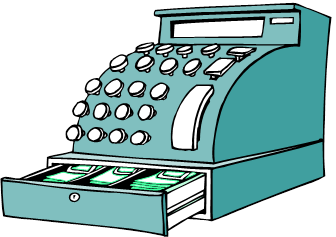Include Top

# DiscoverSim™ Case Studies

## Case Study 1 – Basic Profit Simulation: Introduction

1. This is an example of DiscoverSim Monte Carlo simulation to determine probability of daily profit using a basic profit model for a small retail business. We will apply distribution fitting to historical data and specify input correlations to define the model in a way that closely matches our real world business.

The profit (Pr) requirement is Pr > 0 dollars (i.e., the lower specification limit LSL = 0)

The profit equation, or “Y = f(X) transfer function”, is calculated as follows:

Total Revenue, TR = Quantity Sold * Price

Total Cost, TC = Quantity Sold * Variable Cost + Fixed Cost

Profit, Pr = TR – TC

2.3. In this study we will use DiscoverSim to help us answer the following questions:

1. What is the predicted probability of daily profit?
2. What are the key X variables that influence profit Y? Can we reduce the variation in profit by reducing the variation of the important input variables?

4.  Summary of DiscoverSim Features Demonstrated in Case Study 1: Distribution Fitting – Discrete Batch Fit Distribution Fitting – Continuous Batch Fit Distribution Fitting – Specified Distribution Fit Create Input Distributions with Stored Distribution Fit Specify Input Correlations Run Simulation and display Histograms, Descriptive Statistics, Process Capability Report Percentile Report Scatter Plot/Correlation Matrix Sensitivity Chart of Correlation Coefficients Sensitivity Chart of Regression Coefficients Distribution Fitting – Nonnormal Process Capability

# Web Demos

Our CTO and Co-Founder, John Noguera, regularly hosts free Web Demos featuring SigmaXL and DiscoverSim